Mobile QR Code1. (School of Mechatronics Engineering, Korea University of Technology and Education, 1600, Chungjeol-ro, Byeongcheon-myeon, Dongnam-gu, Cheonan-si, Chungcheongnam-do, 31253, Korea)

Pseudo-resistor, temperature, sensitivity, compensation, amplifier

## I. INTRODUCTION

As a front-end amplifier for measuring a sensor signal, a capacitively coupled instrumentation amplifier (CCIA) for voltage measurement and a transimpedance amplifier (TIA) for current measurement are the most widely used amplifiers. Fig. 1(a) shows the structure of a CCIA with a feedback resistor R$_{f}$ to provide DC bias to the virtual ground nodes (mp and mn) of the amplifier (A$_{c}$). Due to the feedback resistance (R$_{f}$), CCIA has a high-pass filter characteristic and requires a large feedback resistance for low cutoff frequencies. Harrison implemented pseudo-resistor using MOS to realize large feedback resistance in CCIA structure (1). Fig. 1(b) shows the structure of a TIA with a feedback resistor (R$_{f}$) to provide a transimpedance gain from the input current (II$_{in}$) to the output voltage (V$_{out}$). Chuah implemented pseudo-resistor using MOS to realize large feedback resistance in TIA structure (2). The resistors used in these applications range from a few GΩ to a few TΩ. Since it is impossible to implement such high-resistance resistors in an integrated circuit using pure resistor elements, various methods for realizing a pseudo-resistance using a MOS have been introduced (3).

Fig. 1. Applications requiring pseudo-resistors (a) capacitively coupled amplifier and high pass filter characteristics, (b) transimpedance amplifier for current measurement.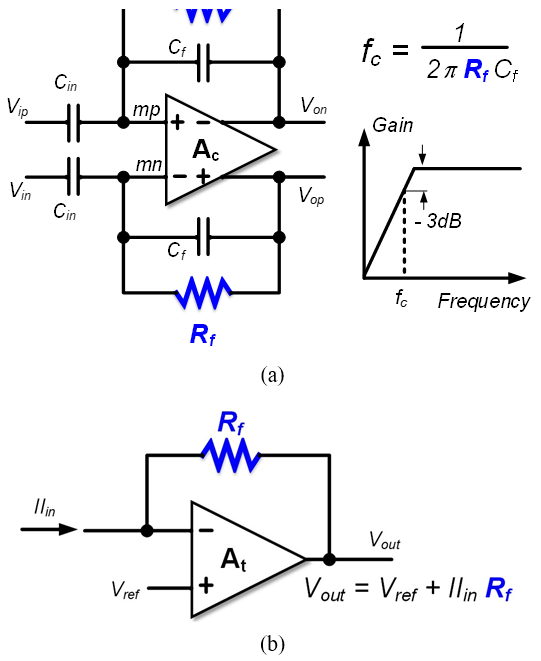Fig. 2. Pseudo-resistor implementation approaches and characteristics (a) passive pseudo-resistor, (b) active pseudo-resistor, (c) detailed structure of active pseudo-resistor, (d) temperature dependency of passive pseudo-resistor, (e) temperature dependency of active pseudo-resistor.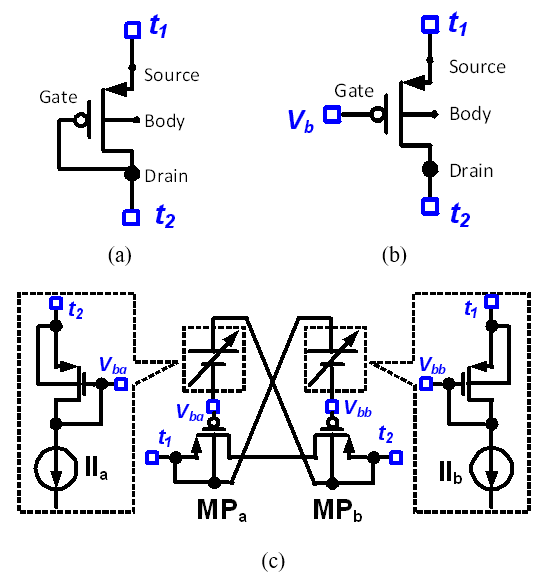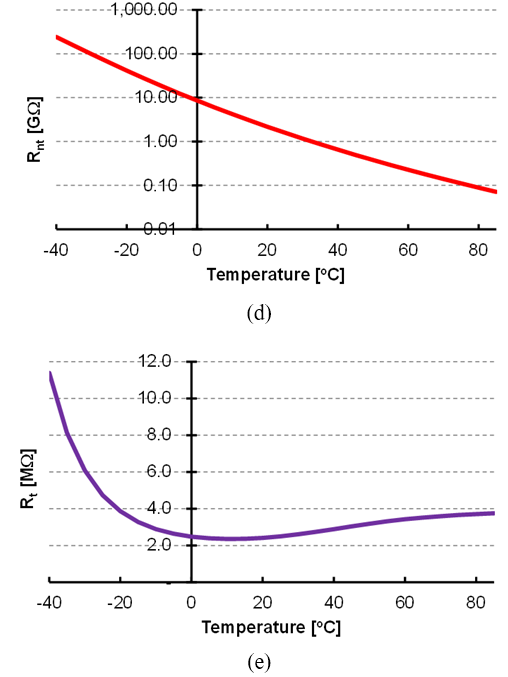Types of pseudo-resistors can be divided into passive pseudo-resistors and active pseudo-resistors. The passive pseudo-resistor has only two terminals (t1 and t2) corresponding to the two terminals of the resistor, as shown in Fig. 2(a), and no additional power-consumed circuit is required. On the other hand, the active pseudo-resistor has a voltage bias terminal (V$_{b}$) added, as shown in Fig. 2(b), and additional power-consumed circuit is required to generate the voltage bias (V$_{b}$). An example of an active pseudo-resistor structure and circuit for generating the bias voltage is shown in Fig. 2(c). When sensor readout circuits are used for industrial products, it must satisfy the specifications for the industrial temperature range of -40 ℃ to 85 ℃. Naturally, the pseudo-resistor must also satisfy the industrial temperature specification. Fig. 2(d) shows the temperature dependence of a passive pseudo-resistor, which has a logarithmic scale variability with respect to temperature variation. In the case of the active pseudo-resistor in Fig. 2(c), the temperature sensitivity is significantly improved compared to the passive pseudo-resistor, but it shows resistance variability of several hundred percent with respect to temperature change. In order for the pseudo-resistor to be used in industrial sensing circuits, the large temperature dependence of the pseudo-resistor must be improved. For this reason, in this paper, a novel pseudo-resistor with improved temperature dependence is proposed.

Section II describes the circuit and operation principle of the newly proposed pseudo-resistor. Section III summarizes the simulation results for the performance of the proposed pseudo-resistor, and Section V concludes.

## II. CIRCUIT DESCRIPTION

Fig. 3(a) shows the circuit structure of the proposed pseudo-resistor. The pseudo-resistor consists of two PMOSs (MP$_{1}$ and MP$_{2}$) and an offset voltage generator (VOFF_GEN). The two PMOSs are symmetrically connected in series and have a potential V$_{mp}$ at a common node. The body bias of each PMOS is all connected to the common node. The VOFF_GEN block receives the voltage V$_{mp}$ from the common node and generates a gate voltage V$_{gp}$ commonly provided to both PMOSs (MP$_{1}$ and MP$_{2}$). The resistance value of the pseudo-resistor can be controlled by adjusting the amount of current flowing through the pseudo-resistor. The current flowing through the pseudo-resistor can be adjusted by controlling the difference voltage (V$_{sg}$ = V$_{mp}$ - V$_{gp}$) between the gate and source of the PMOSs. The VOFF_GEN controls the voltage difference (V$_{sg}$) between the gate and source of the PMOSs. The VOFF_GEN generates the output voltage (V$_{gp}$) by inputting temperature and the voltage (V$_{mp}$) at the common node.

Fig. 3. Detail Circuit structures of (a) pseudo-resistor, (b) offset voltage generator (VOFF_GEN), (c) biasn generator (BIASN_GEN).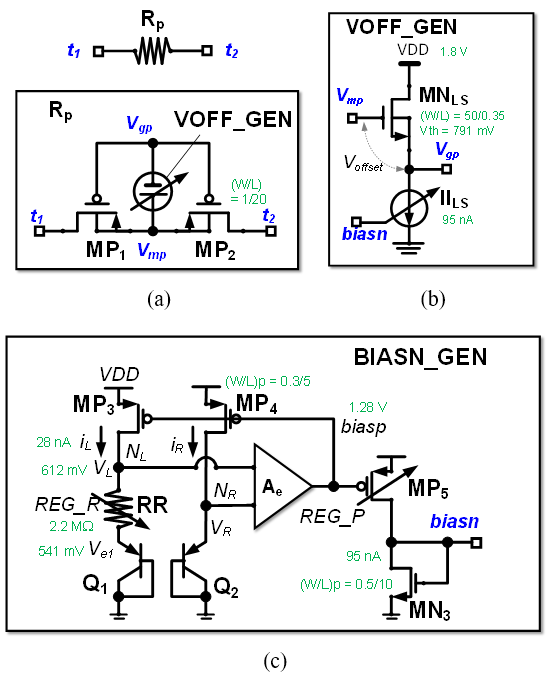Fig. 3(b) shows the detailed structure of the offset generator (VOFF_GEN). The VOFF_GEN is a level shifter (or source follower) composed of one NMOS (MN$_{LS}$) and a current source (II$_{LS}$). The output V$_{gp}$ is generated by being downshifted from the input V$_{mp}$ by the threshold value (V$_{thn}$) of the NMOS (MN$_{LS}$) and current value (II$_{LS}$). The output V$_{gp}$ can be expressed as

##### (1)
$V_{g p}=V_{\operatorname{mp}}-V_{\operatorname{thn}}-\left[\frac{2 I I_{L S}}{\mu_{n} c_{o x}} \cdot\left(\frac{2}{W}\right)_{M N_{L S}}\right]^{0.5}$

in which $\mu_{n}$ is the electron mobility, $C_{o x}$ is the capacitance density of the gate oxide, and $(L / W)_{M N_{L S}}$ is the ratio of gate length to width of MN$_{LS}$. The threshold voltages of the PMOSs (MP$_{1}$ and MP$_{2}$) of $R_p$ and the NMOS (MN$_{LS}$) of VOFF_GEN change according to the temperature change, and as a result, the pseudo-resistance value also changes. The pseudo resistance value change due to temperature change is compensated by the bias current II$_{LS}$. Therefore, the current (II$_{LS}$) must have temperature sensitivity, and the temperature sensitivity must be controllable. The current in the current source (II$_{LS}$) can be tuned by a current control bias (biasn).

Fig. 3(c) shows the detailed structure of the bias generator (BIASN_GEN). The current control bias generation block (BIASN_GEN) produces a control voltage (biasn) used for current control in VOFF_GEN. The current generated by the control voltage (biasn) is proportional to the absolute temperature (PTAT). The PTAT current is generated using parasitic pnp transistors (Q1$_{1}$ and Q1$_{2}$) and resistors (RR). At nodes NL and NR, the currents and voltages are held at the same value ($i_{L}$ = $i_{R}$, V$_{L}$ = V$_{R}$) by the PMOSs (MP$_{3}$ and MP$_{4}$) and the error amplifier (Ae). The emitter voltages (V$_{e1}$ and V$_{R}$) of Q1$_{1}$ and Q1$_{2}$ decrease with increasing temperature. When a voltage having a CTAT(Complementary to absolute temperature) characteristics is applied across the resistor (RR), the current flowing through the resistor (RR) has a PTAT characteristics. The sensitivity to temperature of the PTAT current ($i_{L}$, $i_{R}$) can be adjusted by changing the resistance value (RR). The PTAT current flowing through RR is copied to MN$_{5}$, and the magnitude of the current is scaled to the required level. Eventually, a current controlled bias (biasn) is created in the NMOS (MN$_{3}$).

## III. Simulation Results

The layout of the proposed pseudo-resistor and bias blocks is shown in Fig. 4 with an overall size of 190 µm x 150 µm.

Fig. 5 shows the characteristics of the proposed pseudo-resistor according to the temperature variation from – 40 ℃ to 85 ℃. Fig. 5(a) shows the bias current (II$_{LS}$) in VOFF_GEN according to the temperature variation. PTAT current plots with various slopes and levels can be obtained by adjusting the control registers (REG_RR for RR and REG_P for MN$_{5}$) in Fig. 3(c). Fig. 5(b) shows the change in resistance value according to temperature change at various resistance values. Table 1 summarizes the pseudo-resistance change level with respect to temperature change. For a temperature variation of 125 °C from -40 °C to +85 °C, the pseudo-resistance value has a minimum variation of 1.1 % and a maximum of 2.1 %. Temperature sensitivity (S$_{T}$) can be defined as the rate of change of resistance (RT) for a unit temperature change, and the rate of change of resistance can also be calculated by dividing the maximum variation in resistance ($_{\left(\Delta R_{p}\right)}$) by the median resistance value ($_{\left(\Delta R_{p,mid}\right)}$) as in Eq. (2).

##### (2)
$S_{T}=\frac{R_{T}}{\Delta T}=\frac{\Delta R_{\mathrm{p}}}{R_{p, \operatorname{mid}} \Delta T}$

Fig. 4. Layout of the proposed pseudo-resistor and bias blocks.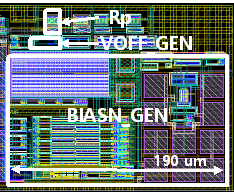Fig. 5. Characteristics of the proposed pseudo-resistor according to the temperature variation (a) PTAT current used in VOFF_GEN, (b) resistance of pseudo-resistor.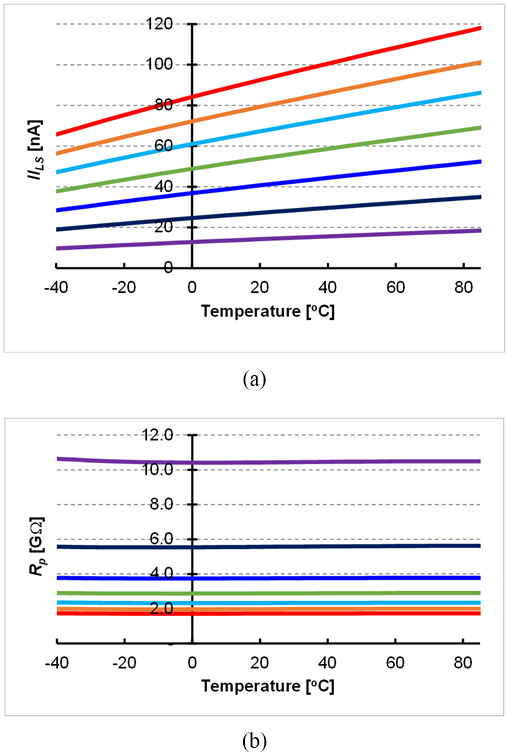Table 1. Resistance value variation of pseudo-resistor ($R_p$) in temperature range(-40℃ ~ 85℃)

 $R_p$ $R_{p,mid}$ [GΩ] $R_{p,max}$ [GΩ] $R_{p,min}$ [GΩ] $\Delta R_{p}$ [%] ST [ppm/℃] Case1 10.5 10.63 10.41 2.1 168 Case2 5.6 5.62 5.53 1.6 128 Case3 3.8 3.78 3.74 1.1 91 Case4 2.9 2.91 2.87 1.2 94 Case5 2.3 2.36 2.32 1.5 120 Case6 2.0 2.00 1.97 1.4 113 Case7 1.7 1.73 1.71 1.2 93

Table 2. Performance summary and comparison

 This work Djekic (4) Process technology 0.18 µm CMOS 0.18 µm CMOS SOI Supply voltage 1.8 V 1.8 V Power consumption 4 uW 200 uW Resistance 1.7 GΩ ~ 10.4 GΩ 10 MΩ ~ 100 MΩ Resistance variation 2.1 % 15 % Temperature range -40 ~ 85 ℃ -40 ~ 125 ℃ Temperature sensitivity 91 ppm/℃ ~ 168 ppm/℃ 900 ppm/℃

For various resistance values from 1.7 GΩ to 10.5 GΩ of pseudo-resistance, the temperature sensitivity has a minimum of 91 ppm/℃ and a maximum of 168 ppm/℃. These results show that the pseudo-resistance maintains a very stable resistance value over a temperature change range of 125 ℃.

Table 1 summarizes the performance indices of the proposed pseudo resistance and compares them with the latest previous work. Compared to previous work, the performance of this paper is superior in terms of temperature sensitivity and power consumption.

## IV. CONCLUSIONS

A novel pseudo-resistor insensitive to temperature change is proposed. The pseudo-resistor is implemented using two PMOSs connected in series and an offset generator (VOFF_GEN) between the gate and source of the PMOSs. The resistance value and temperature sensitivity are controlled by adjusting the voltage difference (V$_{gs}$) between the gate and the source of the PMOS generated by VOFF_GEN. The voltage difference (V$_{gs}$) is generated by the PTAT current (II$_{LS}$), and the magnitude and temperature sensitivity of the PTAT current can be controlled. By adjusting the magnitude of the PTAT current, the pseudo-resistance value could be obtained from 1.7 GΩ to 10.4 GΩ. In addition, by tuning the resistor (RR) that controls the temperature sensitivity of the PTAT current (II$_{LS}$), the temperature sensitivity (S$_{T}$) of the pseudo-resistor was able to obtain a minimum value of 91 ppm/℃ in the range of -40 ℃ to 85 ℃. The proposed pseudo-resistor can be used in many applications with a wide temperature range by being mounted on a charge amplifier and a transimpedance amplifier.

### ACKNOWLEDGMENTS

This work was supported by the National Research Foundation of Korea (NRF) grant funded by the Korea government (MSIT) (No. 2020R1F1A1067128). This paper was supported by the new professor research program of KOREATECH in 2020

### REFERENCES

1
Harrison R., , June, 2003, A Low-Power Low-Noise CMOS Amplifier for Neural Recording Applications, Solid-State Circuits, IEEE Journal of, Vol. 38, No. 6, pp. 958-9652
Chuah J.H., Oct., 2015, Design of Low-Noise High-Gain CMOS Transimpedance Amplifier for Intelligent Sensing of Secondary Electrons, IEEE Sensors Journal, Vol. 15, Vol. 15, No. 10, pp. 5997-60043
Sharma K., June, 2021, MOS based pseudo-resistors exhibiting Tera Ohms of Incremental Resistance for biomedical applications: Analysis and proof of concept, INTEGRAION, the VLSI journal, Vol. 76, pp. 25-394
Djekic D., Nov., 2017, A Transimpedance Amplifier Using a Widely Tunable PVT-Independent Pseudo-Resistor for High-Performance Current Sensing Applications, 43rd IEEE European Solid State Circuits Conference(ESSCIRC), 2017, 11-14, pp. 79-82## Author

received his B.S. degree in mechanical design from the Department of Mechanical Design, Chung-Ang University, Seoul, Korea, M.S. degree in mechanical engi-neering from KAIST, Daejon, Korea, and Ph.D. degrees in electrical engineering and computer science from Seoul National University, Seoul, Korea, in 1995, 1997, and 2003, respectively.

He was a member of research staff at Samsung Advanced Institute of Technology (SAIT) from 2001 to 2019.

In 2020, he joined the Faculty of School of Mechatronics Engineering, Korea University of Technology and Education, Cheonan, Korea.

His research interests include low power and low noise analog integrated circuits for biomedical and MEMS applications.